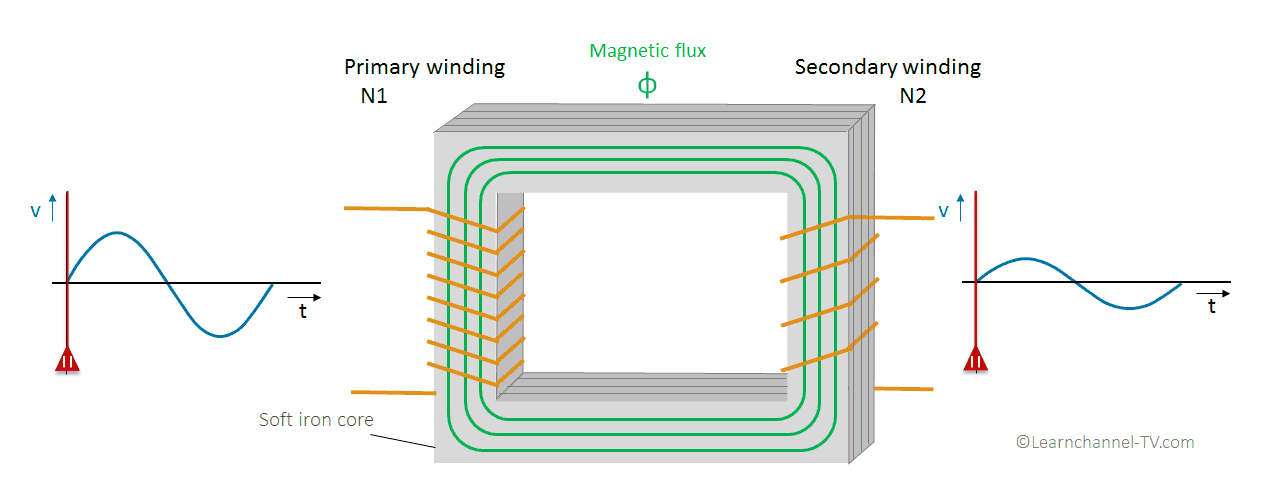## How does a transformer work?Transformer - Function

Now a real test. An isolating transformer is used as feed. The cables are so-called safety cables, i.e. there are no bare ends when disconnected.

By neglecting the losses, the following formulas can be derived:

N1  =   N2            (1)
V1       V2

We verify our assertion with the values from the video:

V2 =   N2  *  V1  =  300  *  100 V  =  25 V
.           N1                  1200

When a load is connected, the output voltage V2 can and will drop. How much it drops depends on the load (output current I2) and how the transformer is designed.

If losses are neglected, however, the output power is equal to the input power:

.           P1              =    P2

=>       U1  *  I1   =   U2  *  I2       (2)

Eq. (1) in eq. (2) shows the relationship between current and number of windings:

I2 =   N1   * I1
.         N2

< Previous Page | Next Page >